Previous: 6. Conclusion and Outlook Up: Dissertation A. Hoessinger Next: Bibliography

# 7. Command-line Interface of MCIMPL

The list of command-line parameters of MCIMPL is available by executing the simulator with the command-line option -help.

MCIMPL -help

The simulation is started by executing

MCIMPL inputName outputName [-option Value]

The required parameters are:

 inputName Name of the file which contains the topological information of the simulation domain in binary PIF-Format ( ). outputName Name of the output file. It is a file in binary PIF-Format which contains the distribution of the implanted particles and the distribution of the material damage. If a three-dimensional simulation is performed the distributions are based on an ortho-grid, in case of a two-dimensional simulation a geometry conforming triangular grid is generated. The output file of a two-dimensional simulation additionally contains the topological information provided on input.

As well the name of the input file as of the output file are strings which may also contain path information according to Unix file system specifications.

In the following all options of the simulator are listed, since all of them are optional the options describing the implantation conditions should be set by the user. The default values for the implantation conditions should just be used for testing the simulator. The options describing the implantation conditions are:
 -ionName -i
Name of the implanted ion species. This name must be contained in the model parameter file mcimpl_data.dat (Sec. 2.2.1). The value of this option is a string. The default value is boron.

 -energy -e
The energy of the implanted ions in keV (Sec. 2.2.2). The value of this option is a single floating point number. The default value is 25.

 -dose -d
The implantation dose in atoms/cm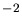(Sec. 2.2.3).The value of this option is a single floating point number. The default value is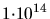.

 -tilt -ti
The angle between the ion beam and the wafer normal (Sec. 2.2.4). The value of this option is a single floating point number. The default value is 0.

 -twist -tw
The rotation angle of the ion beam related to the primary flat of the wafer (Sec. 2.2.4). The value of this option is a single floating point number. The default value is 0.

 -nbIons -nbI
Number of ions used for the simulation. Only the number of ions started from outside of the simulation domain is set by this option. The number of ion trajectories that are generated through the simulation is determined by other parameters or by the applied models (Follow Each Recoil model). The higher the number of simulated ions the higher the accuracy of the simulation results. This number is just an approximate value. The real number of simulated ions is additionally influenced by the size of the simulation domain and the implantation conditions. The value of this option is a single integer number. The default value is 200000. For one-dimensional applications a value of the order of 10000 should be used. For two-dimensional applications about 100000 ions per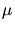m should be used and for three-dimensional applications 2000000 ions perm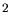are recommended.

Some special process parameters can be defined if necessary:

 -nbRotation -nbRot
One simulation can be performed with several rotation angles (Sec. 4.5.4). This option specifies the number of different rotation angles. The value of this option is a single integer number. The default value is 0, which means that just one ion beam direction is used during the implantation. A value of one equals two ion beam directions with a difference in the rotation angel of 180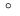.

 -[no]revolving -rev
The wafer is rotated continuously during the simulation. It is a binary option which has no value. By specifying -revolving this option is activated, by specifying -norevolving this option is deactivated. By default this option is deactivated.

 -beamDivergence -beamD
Divergence of the ion beam in(Sec. 4.3). The value of this option is a single floating point number. The default value is 0.

 -crystalOrientation -crystal
This option determines the orientation of the substrate crystal, by defining the miller indices of the crystal direction which is parallel to the z-axis. The x-axis of the simulation domain is always parallel to the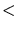100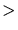direction. The value of this option is a string. Only 100'' or 110'' or 111'' are allowed values. The default value is 100''.

 -temperature -temp
This option determines the wafer temperature, but it has no influence on the simulation behavior, because the lattice vibration is not derived from this temperature but from the option -atomVibration. This option is just used to calculate the critical damage energy which is an output value of the simulator. The value of this option is a single floating point number. The default value is 293.

 -atomVibration -vib
The average amplitude of the thermal lattice vibration in units of the lattice constant (Sec. 3.3.4). The value of this option is a single floating point number. The default value is 0.009.

The following options are used to specify the input and the output of the simulator:

 -inputLogical -inLog
Each physical PIF file may contain several logical files. This option determines the name of the logical file. The value of this option is a string. The default value is MC_In. If the value dose not coincide with any logical file in the physical PIF file the first logical file in the physical PIF file is selected. If the physical PIF file contains just one logical file this option needs not to be defined.

 -outputLogical -outLog
The output PIF file contains one logical file. This option sets the name of this logical file. The value of this option is a string. The default value is MC_Out.

 -[no]damageInput -damIn
Specifies whether the damage information generated by a preceding MCIMPL simulation run shall be reused (Sec. 4.5.1). It is a binary option. By default it is deactivated.

 -[no]pointResponse -pRes
A point response function is calculated which can be used by the analytical ion implantation simulator IMP3D (Sec. 3.2, Sec. 4.5.3). It is a binary option. By default it is deactivated.

 -[no]output1d -out1d
Determines that one file in ASCII format is generated for each impurity species which only represents the z-dependence of the impurity distribution. In case of a one-dimensional simulation this equals the simulation result. In case of higher dimensional simulations it is an average over the other coordinates in space. It is a binary option. By default it is deactivated.

 -backupIntervall -backInt
Determines that a backup file of the current simulation status is generated regularly after a certain amount of ions has been simulated. The name of the backup file equals the name of the output file without the extension .pbf, but with the extension _BCK.DAT. The value of this option is a single integer number. The default value is 50000.

 -[no]useBackup -back
The backup file is used to restart a simulation. It is a binary option. By default it is deactivated.

 -implantationWindow -implWin
If not the whole surface of the simulation domain shall be exposed to the ion implantation simulation a rectangular implantation window can be specified with this option. In case if a point response function is calculated this window has to be determined to identify the position of the significant point and to define the size of the area which is averaged (Sec. 4.2). The value of this option is a list of six floating point numbers, which determine the front left most point (first three values) and the back right most point of the window (last three values) (x1 y1 z1 x2 y2 z2). By default an automatic implantation window is created.

 -[no]useGrid -uGr
In case of a two-dimensional simulation a triangular grid is generated. This options specifies that all points of the grids contained in the input file should be reused for the generation of the grids for the output file. It is a binary option. By default it is deactivated.

 -minGridAngle -minAng
Minimum acceptable angle in a triangular grid element (only relevant for two-dimensional simulations). The value of this option is a single floating point number and the unit is. The default value is 20.0.

 -maxDoseError -maxDo
Maximum acceptable relative dose error in a triangular grid element (only relevant for two-dimensional simulations). The value of this option is a single floating point number. The default value is 0.1.

 -maxGradient -maxGra
Maximum acceptable concentration (logarithmic) gradient in a triangular grid element (only relevant for two-dimensional simulations). The value of this option is a single floating point number. The default value is 3.0.

 -[no]smoothOutput -smooth
A smoothing operation is performed on the simulation results. It is a binary option. By default it is activated.

Several parameters are used to choose between models and to determine additional conditions for models:

 -[no]damage -dam
Determines if material damage is considered during the simulation. It is a binary option. By default it is activated.

 -recoilSpecies -recSp
Defines the recoil species that are handled by the Follow-Each-Recoil method. By default silicon and oxygen are considered (Sec. 3.3.5). The value of this option is a list of stings. Each string determined the name of one atom species in small letters.

 -[no]simpleMolecule -smpl
If simpleMolecule is activated molecular ions are treated as one big ion with a modified damage generation behavior. Just the distribution of one (significant) atom species is calculated (Sec. 4.5.2). It is a binary option. By default it is activated.

 -nbPrimaryIons -nbPrim
Specifies the approximate number of initial ions. For the initial ions no Trajectory-Split method is applied, even if the Trajectory-Split method is enabled. The value of this option is a single integer number. The default value is 0. Care has to be taken that the value is not too high, because this can influence the simulation result. The number of primary ions should not be larger than one hundredth of the total number of simulated ions.

 -splitStrategy -splitStr
The type of Trajectory-Split method which is applied (). The value of this option is a string. Only TRS'' and SRS'' are allowed values. The default value is TRS.

 -oxideThickness -oxide
This option does not influence the behavior of the simulation. The value specified is only written to the point response file for the analytical simulator (only relevant for point response calculation). The analytical simulator can use this parameter as an additional parameter to characterize point response functions. The value of this option is a single floating point number. The default value is 0.0.

 -[no]kinchinPeaseModel -kinch
Determines the damage model used for the simulation. Either the Kinchin-Pease model or the Follow-Each-Recoil model is used. This option is only effective if the use of material damage is enabled. It is a binary option. By default it is activated.

 -simulationDepth -simDepth
In case of a three-dimensional simulation the simulation domain is discretized by an octree. The depth of the root cube in (m) can be set by this option. The value of this option is a single floating point number. The default value is the depth of the simulation domain. Care has to be taken that this value is not smaller than the penetration depth of the ions into the simulation domain. If this is the case some ions get lost.

 -nbOctreeLevel -nbOct
Defines the resolution of the geometry discretization by the octree (only relevant for three-dimensional simulations)  . The value of this option is a single integer number, which may be in the interval from 1 to 10. The default value is 9.

 -nbSplitLevel -nbSpLev
Determines how often one trajectory may be split (Sec. 4.6.1). The value of this option is a single integer number, which may be in the interval from 0 to 10. The default value is 8.

Finally there are some options which set parameters used by physical or empiric models, and which my significantly influence the behavior of the simulator. This options should only be set by expert users when developing new models or when calibrating existing models:

 -finalEnergy -finEng
If the particle energy falls below this limit, the trajectory calculation is stopped. The energy unit is eV. The value of this option is a single floating point number. The default value is 10.0.

 -displacementEnergy -displEng
The energy which is necessary to remove an atom of the target from its lattice position. This displacement energy is applied to all materials and atom species in the simulation domain. The energy unit is eV. The value of this option is a single floating point number. The default value is 15.0.

 -screeningPrefactor -screenPre
Parameter in the empirical electronic stopping model (Sec. 3.3.3). The value of this option is a list of floating point numbers. One value has to be specified for each atom species in the implanted molecule. In case of single atomic ion implantations this list has only one element. The species are ordered according to the order in the chemical formula of the molecule. For instance in case of BF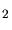ion implantation two floating point numbers have to be defined. The first one is applied to the boron atom and the second one to the fluorine atom. The default values are taken from the file mcimpl_data.dat.

 -lindhardCorrection -lindCorr
Parameter in the empirical electronic stopping model (Sec. 3.3.3). The value of this option is a list of floating point numbers. One value has to be specified for each atom species in the implanted molecule. In case of single atomic ion implantations this list has only one element. The species are ordered according to the order in the chemical formula of the molecule. For instance in case of BFion implantation two floating point numbers have to be defined. The first one is applied to the boron atom and the second one to the fluorine atom. The default values are taken from the file mcimpl_data.dat.

 -nonlocalPrefactor -nlPre
Parameter in the empirical electronic stopping model (Sec. 3.3.3). The value of this option is a list of floating point numbers. One value has to be specified for each atom species in the implanted molecule. In case of single atomic ion implantations this list has only one element. The species are ordered according to the order in the chemical formula of the molecule. For instance in case of BFion implantation two floating point numbers have to be defined. The first one is applied to the boron atom and the second one to the fluorine atom. The default values are taken from the file mcimpl_data.dat.

 -nonlocalPower -nlPow
Parameter in the empirical electronic stopping model (Sec. 3.3.3). The value of this option is a list of floating point numbers. One value has to be specified for each atom species in the implanted molecule. In case of single atomic ion implantations this list has only one element. The species are ordered according to the order in the chemical formula of the molecule. For instance in case of BFion implantation two floating point numbers have to be defined. The first one is applied to the boron atom and the second one to the fluorine atom. The default values are taken from the file mcimpl_data.dat.

 -recombinationFactor -recFac
Parameter in the empirical damage recombination model used in common with the Kinchin-Pease model (Sec. 3.3.5). The value of this option is a list of floating point numbers. One value has to be specified for each atom species in the implanted molecule. In case of single atomic ion implantations this list has only one element. The species are ordered according to the order in the chemical formula of the molecule. For instance in case of BFion implantation two floating point numbers have to be defined. The first one is applied to the boron atom and the second one to the fluorine atom. The default values are taken from the file mcimpl_data.dat.

 -saturation -satur
Parameter in the empirical damage recombination model used in common with the Kinchin-Pease model (Sec. 3.3.5). The value of this option is a list of floating point numbers. One value has to be specified for each atom species in the implanted molecule. In case of single atomic ion implantations this list has only one element. The species are ordered according to the order in the chemical formula of the molecule. For instance in case of BFion implantation two floating point numbers have to be defined. The first one is applied to the boron atom and the second one to the fluorine atom. The default values are taken from the file mcimpl_data.dat.

 -capturingFactor -capt
Parameter in the recombination model used in combination with the Follow Each Recoil method (Sec. 3.3.5). The value of this option is a single floating point number. The unit of this option is the silicon lattice constant. The default value is 1.0.

 -nbConcentrationLevel -nbConc
The Trajectory-Split method uses concentration levels to decide whether an ion is split or not. This options sets the number of these concentration levels. Increasing this number increases the probability of splitting (Sec. 4.6.1). The value of this option is a single integer number in the interval from 1 to 10. The default value is 10.

 -relativeSplitEnergy -relSpEnerg
Sets an energy threshold for the Trajectory-Split method. If the ion energy is above this value no splitting is allowed. It is a relative value to the initial ion energy (Sec. 4.6.1). The value of this option is a single floating point number. The default value is calculated by an empirical formula.

 -relativeSplitLength -relSpLen
The length of the ion trajectory must exceed this value before splitting is allowed. It is relative value to the average trajectory length (Sec. 4.6.1). The value of this option is a single floating point number. The default value is calculated by an empirical formula.

 -searchDistance -searchDis
Parameter which specifies the distance from the ion position, for looking for potential collision partners used by the nuclear and electronic stopping models (Sec. 4.4.1). The value of this option is a single floating point number. The unit is the silicon lattice constant and the default value is 3.0.

 -minScatteringAngle -minScat
This parameter is used when selecting collision partners. If a nuclear scattering event results in a scattering angle above the value specified, the check for collision partners involved in the preceding collision process is omitted when initializing the next stopping process (Sec. 4.4.1). The value of this option is a single floating point number. The unit is degree and the default value is 30.0.

 -minFlightPath -minFlight
If the flight path of a stopping process falls below the value specified the list of lattice sites involved in the stopping process is not replaced by a new list, but the list of the previous stopping process is extended. This list is used to avoid multiple collisions with the same target atoms (Sec. 4.4.1). The value of this option is a single floating point number. The unit is the silicon lattice constant and the default value is 0.05.

A. Hoessiger: Simulation of Ion Implantation for ULSI Technology